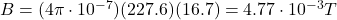## A 15.0 m \long copper wire, 2.20 mm in diameter including insulation, is tightly wrapped in a single layer with adjacent coils touching, to

Question

A 15.0 m \long copper wire, 2.20 mm in diameter including insulation, is tightly wrapped in a single layer with adjacent coils touching, to form a solenoid of diameter 2.10 cm (outer edge).a) What is the length of the solenoid?
b) What is the field at the center when the current in the wire is 16.7 A ?

in progress 0
2 months 2021-07-28T10:45:56+00:00 1 Answers 7 views 0

1. a) 0.5 m

b)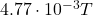Explanation:

a)

At the beginning, the length of the copper wire is:

L = 15.0 m

and the diameter (so, the thickness of each adjacent circle) is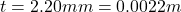While the diameter of one circle of the solenoid is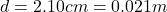So the perimeter of one circle is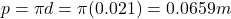So the number of complete circles in the solenoid is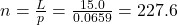The tickness of one circle is, so the total length of the solenoid will be: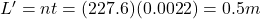b)

The magnetic field at the center of a solenoid is given by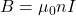where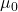is the vacuum permeability

n is the number of turns of the solenoid

I is the current in the solenoid

Here we have: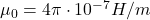is the vacuum permeability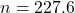is the number of turns in the solenoid (calculated in part a)

I = 16.7 A is the current in the solenoid

Substituting, we find: